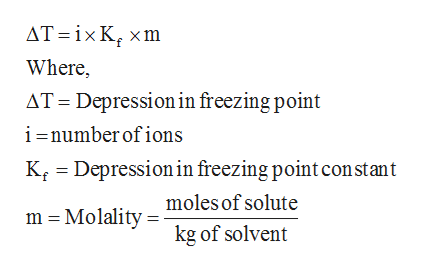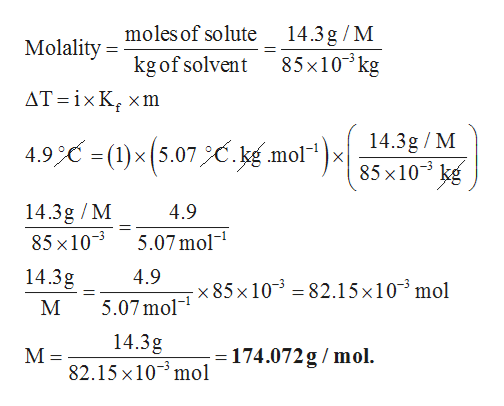# When 14.3 g of a certain molecular compound X are dissolved in 85.0 g of benzene C6H6, the freezing point of the solution is measured to be 0.5 °C. Calculate the molar mass of X. molal freezing point depression constant (Kf)°C·kg·mol−1 of benzen is 5.07 If you need any additional information on benzene, use only what you find in the ALEKS Data resource. Also, be sure your answer has a unit symbol, and is rounded to 2 significant digits.

Question
2 views

When 14.3 g of a certain molecular compound X are dissolved in

85.0 g of benzene C6H6, the freezing point of the solution is measured to be 0.5 °C. Calculate the molar mass of X.

molal freezing point depression constant (Kf)
°C·kg·mol−1 of benzen is 5.07

If you need any additional information on benzene, use only what you find in the ALEKS Data resource. Also, be sure your answer has a unit symbol, and is rounded to

2

significant digits.

check_circle

Step 1

The equation for depression in freezing point is given below.help_outlineImage TranscriptioncloseAT ix K xm Where, AT Depression in freezing point i -number of ions K Depressionin freezing point con stant moles of solute m Molality kg of solvent fullscreen
Step 2

The freezing point of pure benzene is 5.40 C. Then depression in freezing point = freezing point of pure benzene – freezing point of solution = 5.40 C – 0.50 C = 4.90 C. Suppose, the unknown c...help_outlineImage Transcriptionclose14.3g /M 85x103kg moles of solute Molality kgof solvent АТ 3ixK, хm 14.3g / M 85 x 10 kg 4.9= (1)x(5.07C.g mol- X 14.3g /M 85 x103 4.9 5.07mol 14.3g 4.9 x 85 x 103 82.15x103 mol 5.07mol 14.3g М: - 174.072g / mol 82.15 x10 mol fullscreen

### Want to see the full answer?

See Solution

#### Want to see this answer and more?

Solutions are written by subject experts who are available 24/7. Questions are typically answered within 1 hour.*

See Solution
*Response times may vary by subject and question.
Tagged in

### Physical Chemistry